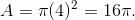Example Questions

Example Question #1 : How To Find Circumference

If a circle has an area of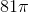, what is the circumference of the circle?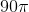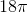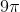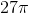Explanation:

The formula for  the area of a circle is πr2. For this particular circle, the area is 81π, so 81π = πr2. Divide both sides by π and we are left with r2=81. Take the square root of both sides to find r=9. The formula for the circumference of the circle is 2πr = 2π(9) = 18π. The correct answer is 18π.

Example Question #1 : How To Find Circumference

A circle with an area of 13π in2 is centered at point C. What is the circumference of this circle?

√26π

√13π

13π

26π

2√13π

2√13π

Explanation:

The formula for the area of a circle is πr2.

We are given the area, and by substitution we know that 13π πr2.

We divide out the π and are left with 13 = r2.

We take the square root of r to find that r = √13.

We find the circumference of the circle with the formula = 2πr.

We then plug in our values to find = 2√13π.

Example Question #1 : How To Find Circumference

A 6 by 8 rectangle is inscribed in a circle. What is the circumference of the circle?

6π

10π

25π

8π

12π

10π

Explanation:

First you must draw the diagram. The diagonal of the rectangle is also the diameter of the circle. The diagonal is the hypotenuse of a multiple of 2 of a 3,4,5 triangle, and therefore is 10.
Circumference = π * diameter = 10π.

Example Question #1 : How To Find Circumference

A gardener wants to build a fence around their garden shown below. How many feet of fencing will they need, if the length of the rectangular side is 12 and the width is 8?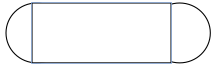96 ft

4π + 24

8π + 24

40 ft.

8π + 24

Explanation:

The shape of the garden consists of a rectangle and two semi-circles. Since they are building a fence we need to find the perimeter. The perimeter of the length of the rectangle is 24. The perimeter or circumference of the circle can be found using the equation C=2π(r), where r= the radius of the circle. Since we have two semi-circles we can find the circumference of one whole circle with a radius of 4, which would be 8π.

Example Question #1 : How To Find Circumference

The diameter of a circle is defined by the two points (2,5) and (4,6). What is the circumference of this circle?

π√2.5

2.5π

π√5

π√5

Explanation:

We first must calculate the distance between these two points. Recall that the distance formula is:√((x2 - x1)2 + (y2 - y1)2)

For us, it is therefore: √((4 - 2)2 + (6 - 5)2) = √((2)2 + (1)2) = √(4 + 1) = √5

If d = √5, the circumference of our circle is πd, or π√5.

Example Question #5 : How To Find Circumference

A car tire has a radius of 18 inches. When the tire has made 200 revolutions, how far has the car gone in feet?

3600π

600π

300π

500π

600π

Explanation:

If the radius is 18 inches, the diameter is 3 feet. The circumference of the tire is therefore 3π by C=d(π). After 200 revolutions, the tire and car have gone 3π x 200 = 600π feet.

Example Question #1 : How To Find Circumference

A circle has the equation below. What is the circumference of the circle?

(x – 2)2 + (y + 3)2 = 9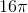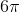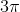Explanation:

The radius is 3. Yielding a circumference of.

Example Question #51 : Circles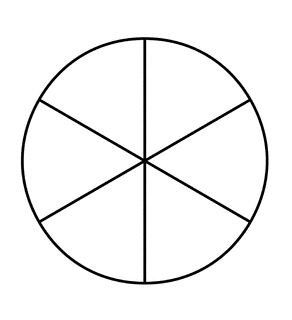If a circle (shown above) with areais divided into 6 equal slices, what is the arc length of one of the slices?

Note: The above figure is not necessarily drawn to scale.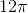Explanation:

Begin by solving for the circumference of the circle. Use the area of the circle, which is given, and the equation for the area of a circle to determine the radius of the circle: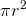=Divide both sides by.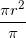=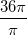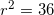Solve for: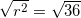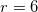The radius of the circle is 6. Now find the circumference.

Circumference is equal to 2 times the radius multiplied by.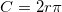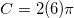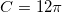Now that we have the circumference, divide by 6 to find the length of one of the slices of the circle: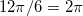The arc length of one of the slices of the circle is.

Example Question #11 : How To Find Circumference

Ashley has a square room in her apartment that measures 81 square feet. What is the circumference of the largest circular area rug that she can fit in the space?Explanation:

In order to solve this question, first calculate the length of each side of the room.The length of each side of the room is also equal to the length of the diameter of the largest circular rug that can fit in the room. Since, the circumference is simplyIf a circle has circumference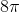, what is its area?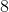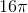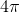If the circumference is, then since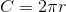we know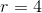. We further know that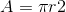, so